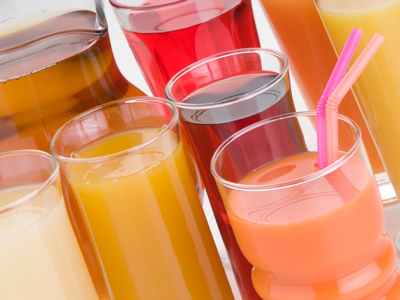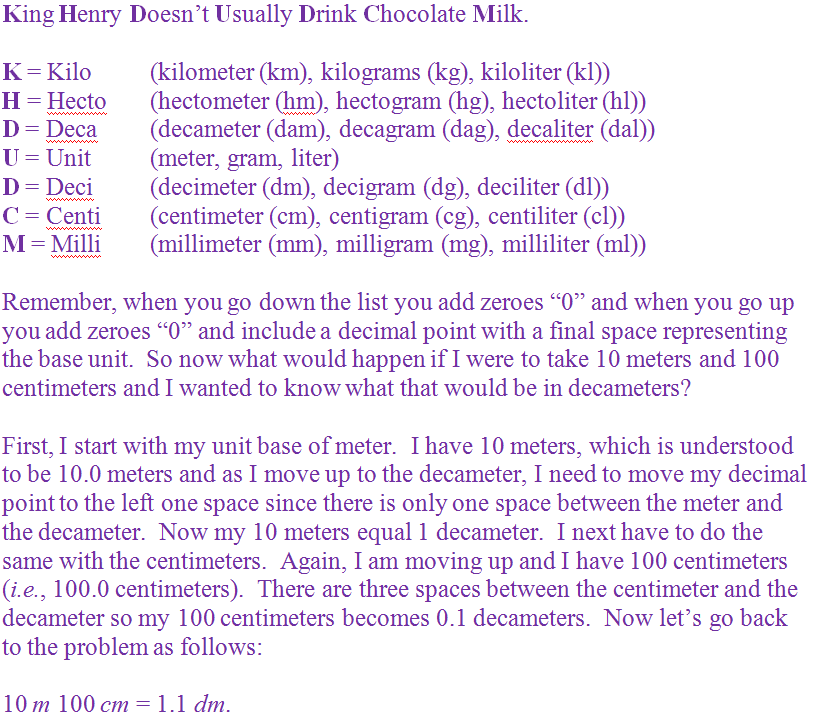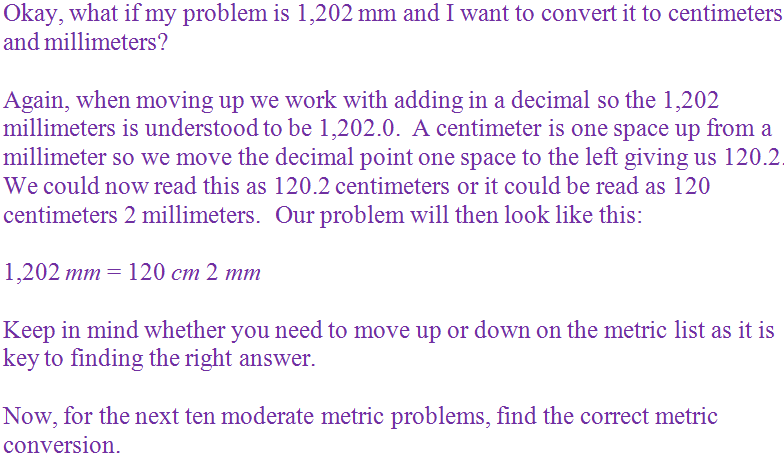Volume is measured in liters.

# Measurement - The Metric System - Moderate Conversions (Part 1)

This Math quiz is called 'Measurement - The Metric System - Moderate Conversions (Part 1)' and it has been written by teachers to help you if you are studying the subject at middle school. Playing educational quizzes is a fabulous way to learn if you are in the 6th, 7th or 8th grade - aged 11 to 14.

It costs only \$12.50 per month to play this quiz and over 3,500 others that help you with your school work. You can subscribe on the page at Join Us

Before tackling this quiz, be sure you are comfortable with knowing the basics of the metric system. Once you have mastered the Easy Conversions, you will be ready to move forward to a little harder conversion or the moderate conversions.

As a quick reminder, the metric system is a form of measurement that works in base 10 conversions. It includes length/distance, mass/weight and volume known as meters, grams and liters.

In order to do the conversions properly, you will need to follow the standard metric listing as shown below.

Go straight to Quiz1.
8,455 ml = ___ l ___ ml
84 l 55 ml
8 l 455 ml
845 l 5 ml
8 l 0.455 ml
As we are moving up from 8,455 milliliters, it is understood that 8,455 milliliters is the same as 8455.0. Moving up we must move the decimal point over one space to the left for each space we go up the metric list. The liter is three spaces up from the milliliter so 8455.0 becomes 8.455. 8,455 milliliters (ml) equals 8.455 liters (l) and/or it equals 8 liters (l) 455 milliliters (ml). Answer (b) is the correct answer
2.
4 kg 329 g = ___ g
4.329 g
43.29 g
432.9 g
4,329 g
As we are moving down the metric list we will be adding zeroes “0”. There are three spaces between a kilogram and a gram so 4 kilograms (kg) equals 4,000 grams (g) plus we have 329 grams (g). 4,000 g + 329 g = 4,329 g. Answer (d) is the correct answer
3.
4,962 g = ___ kg ___ g
4 kg 962 g
49 kg 62 g
496 kg 2 g
49 kg .62 g
As we are moving up from 4,962 grams, it is understood that 4,962 grams is the same as 4962.0. Moving up we must move the decimal point over one space to the left for each space we go up the metric list. The kilogram is three spaces up from the gram so 4962.0 becomes 4.962. 4,962 grams (g) equals 4.962 kilograms (kg) and/or it equals 4 kilograms (kg) 962 grams (g). Answer (a) is the correct answer
4.
7 hm 9m = ___ m
7.09 m
709 m
7,090 m
70,900 m
As we are moving down the metric list we will be adding zeroes “0”. There are two spaces between a hectometer and a meter so 7 hectometers (hm) equals 700 meters (m) plus we have 9 meters (m). 700 m + 9 m = 709 m. Answer (b) is the correct answer
5.
325 cm 44 mm = ___ mm
30,294 mm
3,294 mm
329.4 mm
32.94 mm
As we are moving down the metric list we will be adding zeroes “0”. There is one space between a centimeter and a millimeter so 325 centimeters (cm) equals 3,250 millimeters (mm). 3,250 mm + 44 mm = 3,294 mm. Answer (b) is the correct answer
6.
615 l = ___ hl ___ l
61 hl 5 l
0.6 hl 15 l
6 hl 0.15 l
6 hl 15 l
As we are moving up from 615 liters, it is understood that 615 liters is the same as 615.0. Moving up we must move the decimal point over one space to the left for each space we go up the metric list. The hectoliter is two spaces up from the liter so 615.0 becomes 6.15. 615 liters (l) equals 6.15 hectoliters (hl) and/or it equals 6 hectoliters (hl) 15 liters (l). Answer (d) is the correct answer
7.
488 dal 11 dl = ___ dl
48,811 dl
488,110 dl
4,881,100 dl
4,881.1 dl
As we are moving down the metric list we will be adding zeroes “0”. There are two spaces between a decaliter and a deciliter so 488 decaliters (dal) equals 48,800 deciliters (dl). 48,800 dl + 11 dl = 48,811 dl. Answer (a) is the correct answer
8.
10,218 mg = ___ g ___ mg
1,021 g 8 mg
102 g 18 mg
10 g 218 mg
1 g .0218 mg
As we are moving up from 10,218 milligrams, it is understood that 10,218 milligrams is the same as 10218.0. Moving up we must move the decimal point over one space to the left for each space we go up the metric list. The gram is three spaces up from the milligram so 10218.0 becomes 10.218. 10,218 milligrams (mg) equals 10.218 grams (g) and/or it equals 10 grams (g) 218 milligrams (mg). Answer (c) is the correct answer
9.
27 dm 13 mm = ___ mm
27,130 mm
2,713 mm
271.3 mm
27.13 mm
As we are moving down the metric list we will be adding zeroes “0”. There are two spaces between a decimeter and a millimeter so 27 decimeters (dm) equals 2,700 millimeters (mm). 2,700 mm + 13 mm = 2,713 mm. Answer (b) is the correct answer
10.
34 hg 61 g = ___ g
3,461 g
34,610 g
346,100 g
3,461,000 g
As we are moving down the metric list we will be adding zeroes “0”. There are two spaces between a hectogram and a gram so 34 hectograms (hg) equals 3,400 grams (g) plus we have 61 grams (g). 3,400 g + 61 g = 3,461 g. Answer (a) is the correct answer
Author:  Christine G. Broome